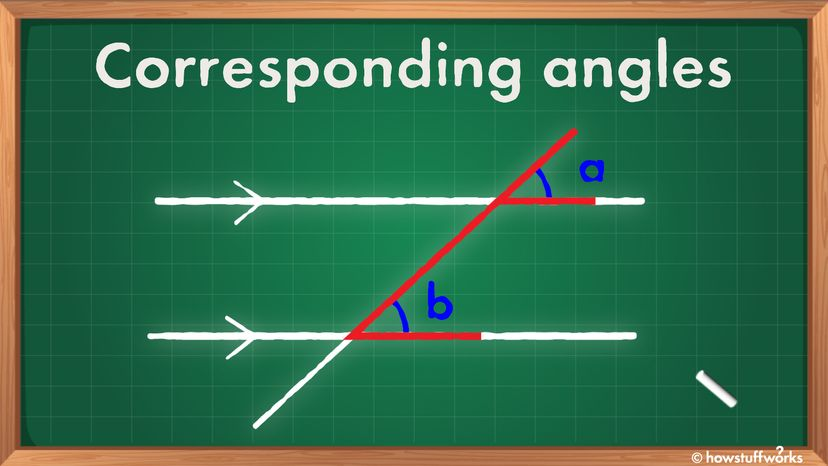# What Are Corresponding Angles and How Do You Find Them?

By: Nathan Chandler  |It's easy to find corresponding angles once you know where to look. HowStuffWorks

Geometry is packed with terminology that precisely describes the way various points, lines, surfaces and other dimensional elements interact with one another. Sometimes they are ridiculously complicated, like rhombicosidodecahedron, which we think has something to do with either "Star Trek" wormholes or polygons. Or how about the 12-sided dodecahedron?

Other times, we're gifted with simpler terms, like corresponding angles.

But before we explain what they are, let's quickly review a few fundamental concepts.

For starters, do you remember the definition of an angle? It's what you get when two rays (lines with a single endpoint) join at a point. The distance between the two rays is the angle.

Parallel lines are two lines on a two-dimensional plane that never cross each other, no matter how long those lines become.

Then, we have transversal lines. This is simply a fancy-pants way of naming a line that crosses at least two other lines.

Now we're getting into the magic. Because when a transversal line crosses two parallel lines, the angles that result from these intersections are very special. That is, the pairs of angles on the same side of the transversal – and in the same position for each line that the transversal crosses – have the same angle. In other words, those angles are congruent (the same).

If that's not clear, maybe the Merriam-Webster definition will help. It says corresponding angles are, "any pair of angles each of which is on the same side of one of two lines cut by a transversal and on the same side of the transversal."

In the main picture above, the corresponding angles are labeled "a" and "b." They have the same angle. You can always find the corresponding angles by looking for the F formation (either forward or backward), highlighted in red. Here is another example in the picture below.In this diagram, line t is the transversal line. Lines a and b are the parallel lines. The angles labeled 1 and 5 are corresponding angles, as are 4 and 8, 2 and 6 and 3 and 7. That means their angles are the same. Jleedev/Wikimedia Commons/CC BY-SA 3.0

John Pauly is a middle school math teacher who uses a variety of ways to explain corresponding angles to his students. He says that many of his students struggle to identify these angles in a diagram.

For instance, he says to take two similar triangles, triangles that are the same shape but not necessarily the same size. these different shapes may be transformed. They may have been resized, rotated or reflected.Here, we see corresponding angles in triangles. The triangles are different, but the same shape, so their corresponding angles are the same. Isipeoria~enwikibooks/Wikimedia Commons/CC BY-SA 3.0

In certain situations, you can assume certain things about corresponding angles.

For instance, take two figures that are similar, meaning they are the same shape but not necessarily the same size. If two figures are similar, their corresponding angles are congruent (the same). That's great, says Pauly, because this allows the figures to keep their same shape.

He says to think of a picture you want to fit into a document. "You know that if you resize the picture you have to pull from a certain corner. If you don't, the corresponding angles won't be congruent, in other words, it will look wonky and out of proportion. This also works for the converse. If you are trying to make a scale model, you know that all of the corresponding angles have to be the same (congruent) in order to get that exact copy you are looking for."